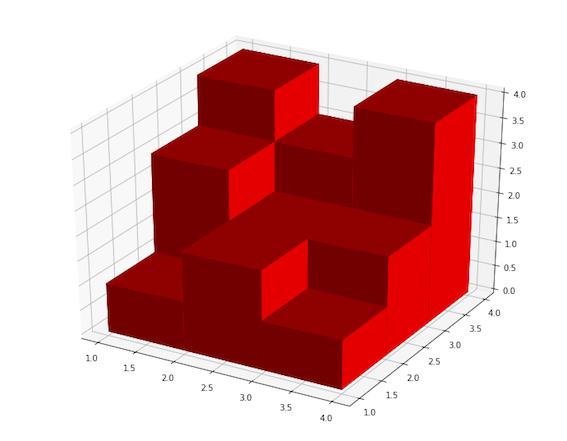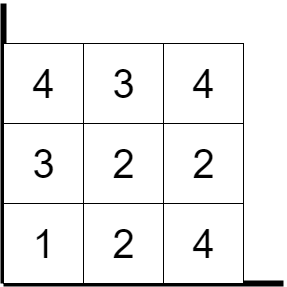Original definition of the problem is given at hackerrank. In order to avoid copyright related issues, I would like to not keep the definition of the problem in here.

# Solution Description

The hint has been read from the discussion list. If the 3d surface made of same-sided cubes then it becomes easy to calculate 3d surface. We have to look at the 3D surface from the top view, that way we can have a 2D surface with height values of each cell.The above 3D object can be converted into 2D form as belowFrom here we have to check the neighbors of the each cell. For example, the cell on top-left has no neighbors on the left and top side, so we can accumulate side surfaces. On the right and bottom, it has neighbors but both of them are smaller than 4. That is why we can accumulate those sides too. Finally, top and bottom surfaces areas are always going to be same H x W, that is why the area can be initialized with sumArea = (H x W x 2)

# Solution Source Code

``````import java.io.*;
import java.math.*;
import java.security.*;
import java.text.*;
import java.util.*;
import java.util.concurrent.*;
import java.util.regex.*;

public class Solution {

// Complete the surfaceArea function below.
static int surfaceArea(int[][] A) {

int H = A.length;
int W = A.length;
System.out.println("H: " + H + " W: " + W);
int area = 2 * (H-2) * (W-2);
for (int i = 1; i < H-1; i++ ) {
for (int j = 1; j < W-1; j++ ) {
//System.out.print(A[i][j] + " ");
area += Math.max(0, A[i][j] - A[i][j-1]);
area += Math.max(0, A[i][j] - A[i][j+1]);
area += Math.max(0, A[i][j] - A[i-1][j]);
area += Math.max(0, A[i][j] - A[i+1][j]);
}
//System.out.println();
}

return area;
}

private static final Scanner scanner = new Scanner(System.in);

public static void main(String[] args) throws IOException {
BufferedWriter bufferedWriter = new BufferedWriter(new FileWriter(System.getenv("OUTPUT_PATH")));

String[] HW = scanner.nextLine().split(" ");

int H = Integer.parseInt(HW);

int W = Integer.parseInt(HW);

int[][] A = new int[H+2][W+2];

for (int i = 1; i <= H; i++) {
String[] ARowItems = scanner.nextLine().split(" ");
scanner.skip("(\r\n|[\n\r\u2028\u2029\u0085])?");

for (int j = 0; j < W; j++) {
int AItem = Integer.parseInt(ARowItems[j]);
A[i][j+1] = AItem;
}
}

int result = surfaceArea(A);

bufferedWriter.write(String.valueOf(result));
bufferedWriter.newLine();

bufferedWriter.close();

scanner.close();
}
}
``````